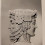## Sunday, April 18, 2010

### Nature by Numbers

I just saw a short video describing nature by numbers. Those who like mathematics may be interested. For those who admire the wonder of god's creation, the video shows that complex structure of living things, such as the shells of Nautilus, the seeds of a Sunflower, and the wings of a dragonfly, can be derived from simple numbers and natural ratio. God just made simple mathematical rules and evolution took over the process of creation.

The video is an animation employing three simple mathematical facts. The first one is the Fibonacci Sequence, named after a thirteenth century Italian mathematician. It is an infinite sequence of natural numbers where the first value is 0, the next is 1 and, from there, each amount is obtained by adding the previous two:

0 1 1 2 3 5 8 13 21 34 55 89 144 233 377 .......

When the sequence is represented in squares, the resulting diagonal curve of each square would join up in a spiral, resembling the complex structure of the shell of a Nautilus.The second simple mathematical fact is the Golden Ratio obtained from the sides of a Golden Rectangle. Splitting from a square, the Golden Rectangle can be drawn with its two sides a and b in a ratio where a/b=(a+b)/a. This constant ratio is an irrational number 1.61803399.... It has a close relationship to the Fibonacci Sequence because the ratio of the two adjacent numbers in the sequence gradually approaches this number:

8/5=1.6
13/8=1.625
21/13=1.615384615
34/21=1.619047619
55/34=1.617647059
55/34=1.617647059
89/55=1.618181818

From the Golden Ratio, the Golden Angle can be derived. It is the angular proportional relationship between two circular segments of length a and b. The value of the angle formed by b is another irrational number, which is rounded to 137.5 degree. This value is deeply present in nature. The video presents an animation on how to configure the structure formed by the sunflower seeds simply by rotating at this angle.

The third segment of the animation works on a mathematical concept called the Voronoi Tessellations. From it, there is a simple process in building many natural structures, like the wings of some insects or these small capillary structure in some plant's leaves.

The process starts with two points and a segment joining them, and then a second orthogonal line right in the middle, which is bisector of the segment. Then a third point is added, generating two new bisectors that intersect with the first. If we continue adding points to generate successive bisectors, their intersections will lead to a series of polygons called Voronoi Tiles around a set of points. The perimeter of each one of these tiles is equidistant to neighboring points and defines their area of influence. All these segments that interconnect the points form a triangular structure called Delaunay Triangulation.

Given a certain number of points in the plane, triangles are drawn using 3 points only if the circumcircle created using these 3 points is empty (not enclosing any other points). The animation then rotates 90 degrees each side of the triangle using the the midpoint after defining the Delaunay Triangulation to construct the Voronoi Tiling. This is how the structure of the dragonfly wing is built.

Now watch the video below to see how these rules are implemented.

1.2.